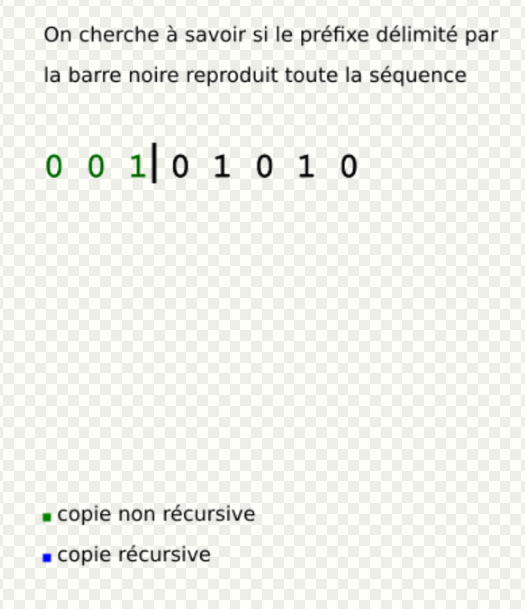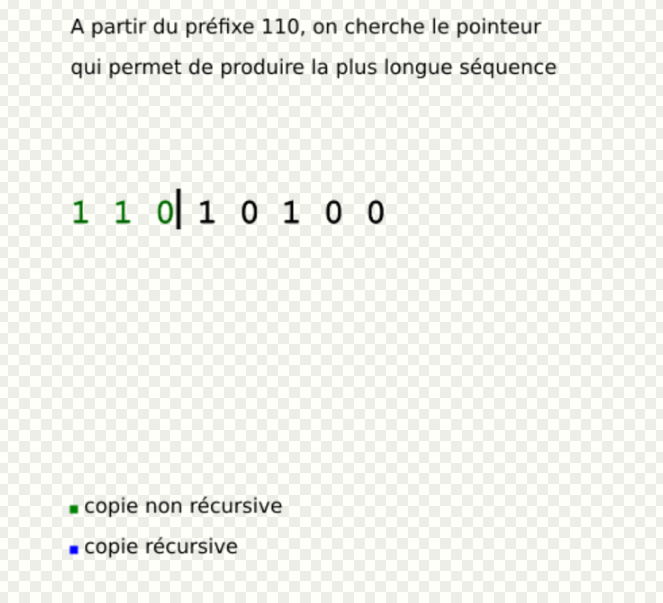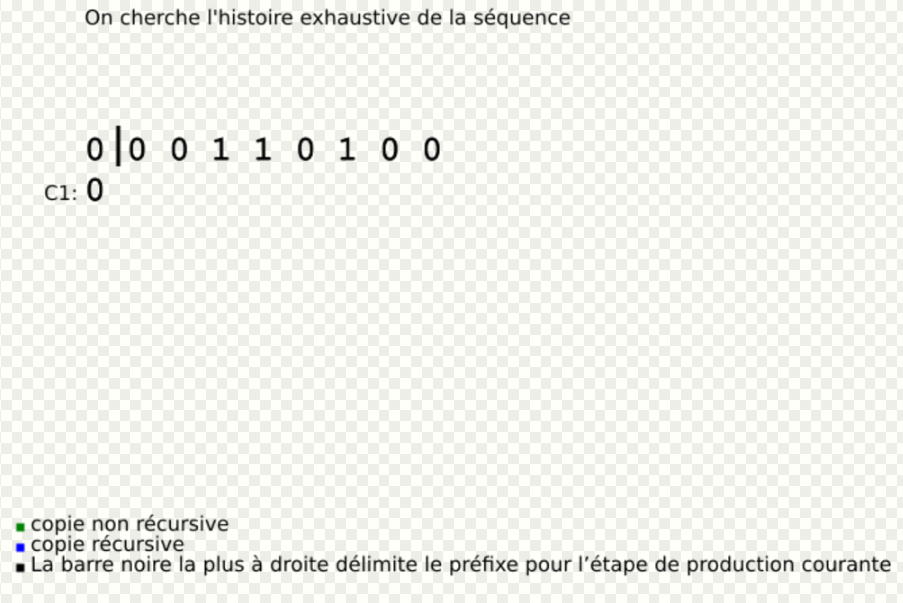Submitted Successfully!
Thank you for your contribution! You can also upload a video entry or images related to this topic.
Ver. Summary Created by Modification Content Size Created at Operation
1 handwiki -- 1192 2022-11-07 01:53:44

Do you have a full video?

# Confirm

Are you sure to Delete?
Cite
Zhu, H. Lempel-Ziv Complexity. Encyclopedia. Available online: https://encyclopedia.pub/entry/33249 (accessed on 09 December 2023).
Zhu H. Lempel-Ziv Complexity. Encyclopedia. Available at: https://encyclopedia.pub/entry/33249. Accessed December 09, 2023.
Zhu, Handwiki. "Lempel-Ziv Complexity" Encyclopedia, https://encyclopedia.pub/entry/33249 (accessed December 09, 2023).
Zhu, H.(2022, November 07). Lempel-Ziv Complexity. In Encyclopedia. https://encyclopedia.pub/entry/33249
Zhu, Handwiki. "Lempel-Ziv Complexity." Encyclopedia. Web. 07 November, 2022.
Lempel-Ziv Complexity

The Lempel-Ziv complexity is a measure that was first presented in the article On the Complexity of Finite Sequences (IEEE Trans. On IT-22,1 1976), by two Israeli computer scientists, Abraham Lempel and Jacob Ziv. This complexity measure is related to Kolmogorov complexity, but the only function it uses is the recursive copy (i.e., the shallow copy). The underlying mechanism in this complexity measure is the starting point for some algorithms for lossless data compression, like LZ77, LZ78 and LZW. Even though it is based on an elementary principle of words copying, this complexity measure is not too restrictive in the sense that it satisfies the main qualities expected by such a measure: sequences with a certain regularity do not have a too large complexity, and the complexity grows as the sequence grows in length and irregularity. The Lempel-Ziv complexity can be used to measure the repetitiveness of binary sequences and text, like song lyrics or prose. Fractal dimension estimates of real-world data have also been shown to correlate with Lempel-Ziv complexity.

fractal real-world data binary sequences

## 1. Principle

Let S be a binary sequence, of length n, for which we have to compute the Lempel-Ziv complexity, denoted C(S). The sequence is read from the left.

Imagine you have a delimiting line, which can be moved in the sequence during the calculation. At first, this line is set just after the first symbol, at the beginning of the sequence. This initial position is called position 1, from where we have to move it to a position 2, which is considered the initial position for the next step (and so on). We have to move the delimiter (starting in position 1) the further possible to the right, so that the sub-word between position 1 and the delimiter position be a word of the sequence that starts before the position 1 of the delimiter.

As soon as the delimiter is set on a position where this condition is not met, we stop, move the delimiter to this position, and start again by marking this position as a new initial position (i.e., position 1). Keep iterating until the end of the sequence. The Lempel-Ziv complexity corresponds to the number of iterations needed to finish this procedure.

Said differently, the Lempel-Ziv complexity is the number of different sub-strings (or sub-words) encountered as the binary sequence is viewed as a stream (from left to right).

## 2. Formal Explanations

The method proposed by Lempel and Ziv uses three notions: reproducibility, producibility and exhaustive history of a sequence, that we defined here.

### 2.1. Notations

Let S be a binary sequence of length n (i.e., n symbols taking value 0 or 1). Let S(i,j), with $\displaystyle{ 1\leq i,j\leq n }$, be the sub-word of S from index i to index j (if j<i, S(i,j) is the empty string). The length n of S is denoted l(S), and a sequence Q is said to be a fixed prefix of S if:

$\displaystyle{ \exists j\lt {\text{l(S), s.t. S(1,j) = Q .}} }$

### 2.2. Reproducibility and ProducibilityExample of reproducibility. https://handwiki.org/wiki/index.php?curid=2061090

On the one hand, a sequence S of length n is said to be reproducible from its prefix S(1,j) when S(j+1,n) is a sub-word of S(1,n-1). This is denoted S(1,j)→S.

Said differently, S is reproducible from its prefix S(1,j) if the rest of the sequence, S(j+1,n), is nothing but a copy of another sub-word (starting at an index i < j+1) of S(1,n-1).

To prove that the sequence S can be reproduced by one of its prefix S(1,j), you have to show that:

$\displaystyle{ \exists p\leq j, {\text{ s.t. }}S(j+1,n)=S(p,l(S(j+1,n))+p-1) }$Example of Productibility. https://handwiki.org/wiki/index.php?curid=2011646

On the other hand, the producibility, is defined from the reproducibility : a sequence S is producible from its prefix S(1,j) if S(1,n-1) is reproducible from S(1,j). This is denoted S(1,j)⇒S. Said differently, S(j+1,n-1) has to be a copy of another sub-word of S(1,n-2). The last symbol of S can be a new symbol (but could not be), possibly leading to the production of a new sub-word (hence the term producibility).Comparison between reproducibility and producibility. https://handwiki.org/wiki/index.php?curid=2031092

### 2.3. Exhaustive History and Complexity

From the definition of productibility, the empty string Λ=S(1,0) ⇒ S(1,1). So by a recursive production process, at step i we have S(1,hi) ⇒ S(1,hi+1), so we can build S from its prefixes. And as S(1,i) ⇒ S(1,i+1) (with hi+1 =hi + 1) is always true, this production process of S takes at most n=l(S) steps. Let m, $\displaystyle{ 1\leq {\text{m}}\leq l(S) }$, be the number of steps necessary for this product process of S. S can be written in a decomposed form, called history of S, and denoted H(S), defined like this:

$\displaystyle{ H(S)=S(1,h_{1})S(h_{1}+1,h_{2})\dotsm S(h_{{m-1}}+1,h_{m}) }$ $\displaystyle{ H_{i}(S)=S(h_{{i-1}}+1,h_{i}),i=1,2\dotsm m, {\text{where} }\; h_{0}=0,h_{1}=1,h_{m}=l(S),{\text{ is called component of } H(S)}. }$

A component of S, Hi(S), is said to be exhaustive if S(1,hi) is the longest sequence produced by S(1,hi-1) (i.e., S(1,hi-1) ⇒ S(1,hi)) but so that S(1,hi-1) does not produce S(1,hi) (denoted ).$\displaystyle{ S(1,h_{i}-1)\nrightarrow S(1,h_{i}) }$ The index p which allows to have the longest production is called pointer.

The history of S is said to be exhaustive if all its component are exhaustive, except possibly the last one. From the definition, one can show that any sequence S has only one exhaustive history, and this history is the one with the smallest number of component from all the possible histories of S. Finally, the number of component of this unique exhaustive history of S is called the Lempel-Ziv complexity of S.

## 3. Algorithm

Fortunately, there exists a very efficient method for computing this complexity, in a linear number of operation ($\displaystyle{ \mathcal{O}(n) }$ for $\displaystyle{ n=l(S) }$ length of the sequence S).

A formal description of this method is given by the following algorithm:

• i = p - 1, p is the pointer (see above)
• u is the length of the current prefix
• v is the length of the current component for the current pointer p
• vmax is the final length used for the current component (largest on all the possible pointers p)
• and C is the Lempel-Ziv Complexity, incremented iteratively.
// S is a binary sequence of size n i := 0 C := 1 u := 1 v := 1 vmax := v while u + v <= n do if S[i + v] = S[u + v] then v := v + 1 else vmax := max(v, vmax) i := i + 1 if i = u then // all the pointers have been treated C := C + 1 u := u + vmax v := 1 i := 0 vmax := v else v := 1 end if end if end while if v != 1 then C := C+1 end if
Information
Subjects:
Contributor MDPI registered users' name will be linked to their SciProfiles pages. To register with us, please refer to https://encyclopedia.pub/register :
View Times: 286
Entry Collection:
Revision: 1 time (View History)
Update Date: 07 Nov 2022
1000/1000